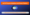# GATE Physics Syllabus 2021 and Exam PatternGATE Physics Syllabus: Physics is the natural science that studies matter and its motion and behavior through space and time and that studies the related entities of energy and force.

You must aware of the latest GATE Physics syllabus and marking scheme to prepare for the Physics exam. To prepare for the GATE Physics exam, you should know the latest ECE Syllabus and marking scheme.

Here we are providing you the complete guide on GATE Physics Syllabus 2021 and Marking Scheme.

## GATE Physics Syllabus 2021

In the latest GATE Syllabus for Physics, you will find the important chapters and concepts to be covered in all subjects. GATE 2021 Physics syllabus will have core physics and general aptitude topics. Mainly the questions will be asked from core Physics and the rest of the questions will be asked from general aptitude topics.

After qualifying GATE 2021, candidates will be able to get admission in M.tech courses offered at various institutes of the country like IITs, NITs, etc. You can start your exam preparation by creating a solid study plan and score a better mark in the exam with the latest Physics Syllabus. Based on the score in GATE Physics, you can shape your career in the proper way.

You must have Physics books & study materials, Previous years questions paper along with the latest Physics Syllabus to enhance your semester exam preparation.

Check the complete GATE Physics syllabus below.

### Section 1: Mathematical Physics

• Linear vector space: basis, orthogonality and completeness; matrices; vector calculus; linear differential equations; elements of complex analysis: Cauchy Riemann conditions, Cauchy’s theorems, singularities, residue theorem and applications; Laplace transforms, Fourier analysis; elementary ideas about tensors: covariant and contravariant tensor, Levi-Civita and Christoffel symbols.

### Section 2: Classical Mechanics

• Alembert’s principle, cyclic coordinates, variational principle, Lagrange’s equation of motion, central force and scattering problems, rigid body motion; small oscillations, Hamilton’s formalisms; Poisson bracket; special theory of relativity: Lorentz transformations, relativistic kinematics, mass‐energy equivalence.

### Section 3: Electromagnetic Theory

• Solutions of electrostatic and magnetostatic problems including boundary value problems; dielectrics and conductors; Maxwell’s equations; scalar and vector potentials; Coulomb and Lorentz gauges; Electromagnetic waves and their reflection, refraction, interference, diffraction and polarization; Poynting vector, Poynting theorem, energy and momentum of electromagnetic waves; radiation from a moving charge.

### Section 4: Quantum Mechanics

• Postulates of quantum mechanics; uncertainty principle; Schrodinger equation; one-, two- and three-dimensional potential problems; particle in a box, transmission through one dimensional potential barriers, harmonic oscillator, hydrogen atom; linear vectors and operators in Hilbert space; angular momentum and spin; addition of angular momenta; time independent perturbation theory; elementary scattering theory.

### Section 5: Thermodynamics and Statistical Physics

• Laws of thermodynamics; macrostates and microstates; phase space; ensembles; partition function, free energy, calculation of thermodynamic quantities; classical and quantum statistics; degenerate Fermi gas; black body radiation and Planck’s distribution law; Bose‐Einstein condensation; first and second order phase transitions, phase equilibria, critical point.

### Section 6: Atomic and Molecular Physics

• Spectra of one‐ and many‐electron atoms; LS and jj coupling; hyperfine structure; Zeeman and Stark effects; electric dipole transitions and selection rules; rotational and vibrational spectra of diatomic molecules; electronic transition in diatomic molecules, Franck‐Condon principle; Raman effect; NMR, ESR, X-ray spectra; lasers: Einstein coefficients, population inversion, two and three level systems.

### Section 7: Solid State Physics & Electronics

• Elements of crystallography; diffraction methods for structure determination; bonding in solids; lattice vibrations and thermal properties of solids; free electron theory; band theory of solids: nearly free electron and tight binding models; metals, semiconductors and insulators; conductivity, mobility and effective mass; optical, dielectric and magnetic properties of solids; elements of superconductivity: Type-I and Type II superconductors, Meissner effect, London equation. Semiconductor devices: diodes, Bipolar Junction Transistors, Field Effect Transistors; operational amplifiers: negative feedback circuits, active filters and oscillators; regulated power supplies; basic digital logic circuits, sequential circuits, flip‐flops, counters, registers, A/D and D/A conversion.

### Section 8: Nuclear and Particle Physics

• Nuclear radii and charge distributions, nuclear binding energy, Electric and magnetic moments; nuclear models, liquid drop model: semi‐empirical mass formula, Fermi gas model of nucleus, nuclear shell model; nuclear force and two nucleon problem; alpha decay, beta‐decay, electromagnetic transitions in nuclei; Rutherford scattering, nuclear reactions, conservation laws; fission and fusion; particle accelerators and detectors; elementary particles, photons, baryons, mesons and leptons; quark model.

## GATE 2021 Exam Pattern of Physics

Section  Distribution of Marks Total Marks Types of questions
General Ability 5 questions of 1 mark each
5 questions of 2 marks each

15 marks

MCQs
Physics 25 questions of 1 mark each
30 questions of 2 marks each
85 marks MCQs and NATs

#### Marking scheme

• Total marks: 100 marks
• Negative marking: Only for the MCQs
Type of question Negative marking for wrong answer
MCQs

1/3 for 1 mark questions

2/3 for 2 marks questions

NATs No negative marking

Now you have a detailed guide on GATE Physics Syllabus 2021. If you have any questions related to GATE Physics Syllabus, please let us know in the comment section.